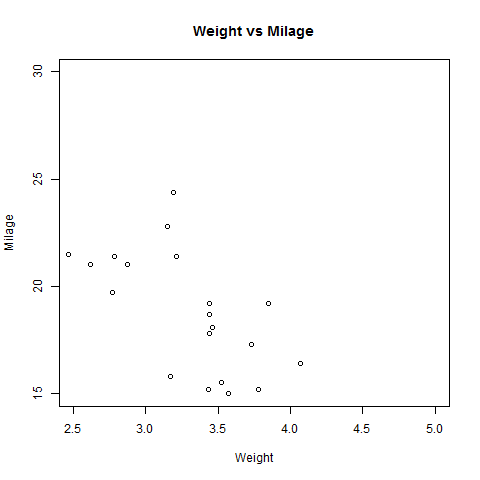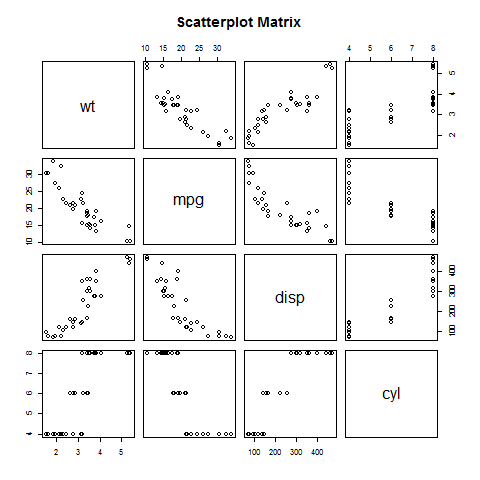# R Visualisation for Beginners – Scatterplots## R Visualisation for Beginners – Scatterplots

Scatterplots show many points plotted in the Cartesian plane. Each point represents the values of two variables. One variable is chosen in the horizontal axis and another in the vertical axis.

The simple scatterplot is created using the plot() function.

### Syntax

The basic syntax for creating scatterplot in R is −

```plot(x, y, main, xlab, ylab, xlim, ylim, axes)
```

Following is the description of the parameters used −

• x is the data set whose values are the horizontal coordinates.
• y is the data set whose values are the vertical coordinates.
• main is the tile of the graph.
• xlab is the label in the horizontal axis.
• ylab is the label in the vertical axis.
• xlim is the limits of the values of x used for plotting.
• ylim is the limits of the values of y used for plotting.
• axes indicates whether both axes should be drawn on the plot.

### Example

We use the data set “mtcars” available in the R environment to create a basic scatterplot. Let’s use the columns “wt” and “mpg” in mtcars.

```input <- mtcars[,c('wt','mpg')]

When we execute the above code, it produces the following result −

```                    wt      mpg
Mazda RX4           2.620   21.0
Mazda RX4 Wag       2.875   21.0
Datsun 710          2.320   22.8
Hornet 4 Drive      3.215   21.4
Valiant             3.460   18.1
```

## Creating the Scatterplot

The below script will create a scatterplot graph for the relation between wt(weight) and mpg(miles per gallon).

```# Get the input values.
input <- mtcars[,c('wt','mpg')]

# Give the chart file a name.
png(file = "scatterplot.png")

# Plot the chart for cars with weight between 2.5 to 5 and mileage between 15 and 30.
plot(x = input\$wt,y = input\$mpg,
xlab = "Weight",
ylab = "Milage",
xlim = c(2.5,5),
ylim = c(15,30),
main = "Weight vs Milage"
)

# Save the file.
dev.off()```

When we execute the above code, it produces the following result −## Scatterplot Matrices

When we have more than two variables and we want to find the correlation between one variable versus the remaining ones we use scatterplot matrix. We use pairs() function to create matrices of scatterplots.

### Syntax

The basic syntax for creating scatterplot matrices in R is −

```pairs(formula, data)
```

Following is the description of the parameters used −

• formula represents the series of variables used in pairs.
• data represents the data set from which the variables will be taken.

### Example

Each variable is paired up with each of the remaining variable. A scatterplot is plotted for each pair.

```# Give the chart file a name.
png(file = "scatterplot_matrices.png")

# Plot the matrices between 4 variables giving 12 plots.

# One variable with 3 others and total 4 variables.

pairs(~wt+mpg+disp+cyl,data = mtcars,
main = "Scatterplot Matrix")

# Save the file.
dev.off()```

When the above code is executed we get the following output.Scatter Matrix Plots | Jupyter Notebook | Python Data Science for beginners

# Personal Career & Learning Guide for Data Analyst, Data Engineer and Data Scientist

## Applied Machine Learning & Data Science Projects and Coding Recipes for Beginners

A list of FREE programming examples together with eTutorials & eBooks @ SETScholars

# Projects and Coding Recipes, eTutorials and eBooks: The best All-in-One resources for Data Analyst, Data Scientist, Machine Learning Engineer and Software Developer

Topics included: Classification, Clustering, Regression, Forecasting, Algorithms, Data Structures, Data Analytics & Data Science, Deep Learning, Machine Learning, Programming Languages and Software Tools & Packages.
(Discount is valid for limited time only)`Disclaimer: The information and code presented within this recipe/tutorial is only for educational and coaching purposes for beginners and developers. Anyone can practice and apply the recipe/tutorial presented here, but the reader is taking full responsibility for his/her actions. The author (content curator) of this recipe (code / program) has made every effort to ensure the accuracy of the information was correct at time of publication. The author (content curator) does not assume and hereby disclaims any liability to any party for any loss, damage, or disruption caused by errors or omissions, whether such errors or omissions result from accident, negligence, or any other cause. The information presented here could also be found in public knowledge domains.`

# Learn by Coding: v-Tutorials on Applied Machine Learning and Data Science for Beginners

Please do not waste your valuable time by watching videos, rather use end-to-end (Python and R) recipes from Professional Data Scientists to practice coding, and land the most demandable jobs in the fields of Predictive analytics & AI (Machine Learning and Data Science).

The objective is to guide the developers & analysts to “Learn how to Code” for Applied AI using end-to-end coding solutions, and unlock the world of opportunities!